# TutorialSelecting the correct altitude for a given peak hull temp with the Ravenstar.

#### dgatsoulis

##### ele2png user
Before we start I'd like to thank Douglas E. Beachy and Steve "Coolhand" Tyler for creating one of the most amazing and fun to fly spacecrafts in Orbiter. Also an additional thanks to Doug for sharing a part of the Ravenstar's hull temperature code with us, without which this tutorial wouldn't be possible.

So, let's begin:

One of the most important factors in a successful aerobrake/aerocapture maneuver with an XR2 is the periapsis altitude of the trajectory. Especially when you are approaching a planet with a high velocity. Too low and you'll burn up; too high and you won't get captured by the atmosphere. Many rely on experience to help them select the correct altitude at which to perform the aerocapture, but even the most experienced -every once in a while- come by a situation where they don't really know what to do.

This tutorial will show you how to calculate in advance the lowest altitude that you can dive in an atmosphere, for a given hull temperature.

The Ravenstar uses the following to calculate the hull temperature:
XR2 nose temp in Kelvin = effectiveOAT + ((1.4 * 3.1034e-10 * 0.642) * ((atmpressure / 2) * (airspeed^3)))
[Note: effectiveOAT, "Effective Outside Air Temperature", is computed from GetAtmTemperature() from the core, but of course the affect it has on hull temperature is only a factor at all where there is enough static pressure to make any difference. The effect it has varies by static pressure.

This is only for the nose hull temp, but it's the only one we need, since it's the highest temperature during an aerobrake. It is safe to assume that OAT is negligable during the initial part of the aerobrake.

The atmospheric pressure is directly linked to the altitude. For most of the planets we can find the pressure for a given altitude by: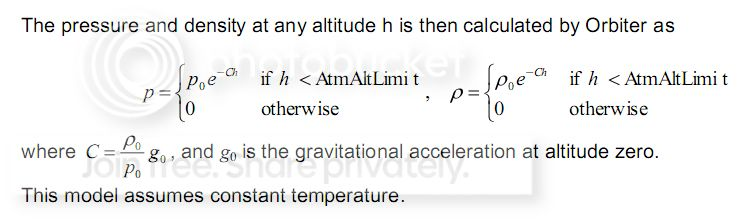(from Orbitersdk\doc\API_guide.pdf)

Earth, Venus and Mars however, have custom atmospheric models, so we need a table with the static pressure at a range of altitudes that correspond to typical reentries.

It is pretty easy to make one:
-Run a scenario in Orbiter.
-Pause
-Scenario Editor
-Bring in a DG and place it at the planet/altitude you want.
-Unpause and pause again (really quick, it's better to be at x0.1 time accel)
-Make a note of the STP from the Surface MFD
-Repeat the last three steps for the range of altitudes you want.

Here is a table for the static pressures of all 3 planets at a range of altitudes that correspond to typical (and some not so typical) aerobrake maneuvers:

Planet|Earth
ALT(km)|Pressure (Pa)
45| 149.095894
46| 131.335401
47| 115.846202
48| 102.290944
49| 90.332336
50| 79.774900
51| 70.454025
52| 62.585778
53| 54.870070
54| 48.334190
55| 42.521857
56| 37.359170
57| 32.779092
58| 28.720982
59| 25.129928
60| 21.956319
61| 19.155356
62| 16.686657
63| 14.513831
64| 12.604141
65| 10.928158
66| 9.459474
67| 8.174416
68| 7.051774
69| 6.072592
70| 5.219932
71| 4.492472
72| 3.832674
73| 3.279521
74| 2.800236
75| 2.387590
76| 2.032800
77| 1.728172
78| 1.466983
79| 1.243359
80| 1.052175
81| 0.888969
82| 0.749858
83| 0.631467
84| 0.530869
85| 0.445528
|
Planet|Mars
ALT(km)|Pressure (Pa)
10| 223.444645
11| 201.211149
12| 180.997468
13| 162.637829
14| 145.978606
15| 130.947896
16| 117.471722
17| 105.389143
18| 94.555333
19| 84.840626
20| 76.128859
21| 68.292238
22| 61.211232
23| 54.817611
24| 49.049231
25| 43.849155
26| 39.165244
27| 34.949778
28| 31.159137
29| 27.753443
30| 24.696293
31| 21.960625
32| 19.528635
33| 17.367162
34| 15.445985
35| 13.738269
36| 12.220198
37| 10.870614
38| 9.670737
39| 8.603887
40| 7.655251
41| 6.811672
42| 6.061465
43| 5.394249
44| 4.800802
45| 4.272934
46| 3.803365
47| 3.385628
48| 3.013976
49| 2.683303
50| 2.389071
|
Planet|Venus
ALT(km)|Pressure (Pa)
70| 2,174.121143
71| 1,780.922039
72| 1,456.860914
73| 1,189.260173
74| 968.728835
75| 787.360326
76| 638.513600
77| 516.618107
78| 417.013700
79| 335.806226
80| 269.749596
81| 216.143410
82| 172.745445
83| 137.697998
84| 109.465603
85| 86.781718
86| 68.604082
87| 54.076894
88| 42.499266
89| 33.298619
90| 26.008301
91| 20.248931
92| 15.724136
93| 12.208767
94| 9.480090
95| 7.361888
96| 5.717444
97| 4.440682
98| 3.449321
99| 2.679496
100| 2.081652
101| 1.617331
102| 1.256682
103| 0.976534
104| 0.758901
105| 0.589818
106| 0.458444
107| 0.356362
108| 0.277032
109| 0.215380
110| 0.167462

Let's re-arrange the hull temp equation to find the static pressure.

$Stat.Pressure=\frac{HullTemp(K)}{1.39466796 \cdot 10^{-10}\cdot Airspeed^3}$
The maximum nose hull temperature for the XR2 is 2840°C. I don't recommend going higher than 2500°C, since any mistake near those temperatures will result in the loss of crew and ship.
So for the hull temp we can use 2500°C = 2773.15°K

All we need to know is the airspeed at periapsis, calculate the static pressure and look up the table to find the altitude.

Here we need to remember that the hull temp is calculated using the airspeed, not the orbital velocity. For Venus, this doesn't pose much of a problem, since it rotates so slowly, but for Earth, you can have a difference of up to +/- 464 m/s depending on the direction of your trajectory at periapsis. For Mars the difference can be up to +/- 240 m/s. Since the airspeed is raised to the power of 3, such an error may have a big impact in our hull temp calculation.

In Orbiter, the atmosphere rotates with the planet, so we can easily calculate the airspeed at periapsis, if we know the orbital velocity, latitude and heading at periapsis (all given by IMFD's Map program)

Airspeed(Pe) = $\sqrt{PeV^2 + (EqRot \cdot cos(lat))^2 - 2 \cdot PeV \cdot (EqRot \cdot cos(lat)) \cdot cos(90-heading)}$Where PeV = the periapsis orbital velocity, EqRot = planet's rotation velocity at the equator = 2*π*Radius/siderial day, lat = geographical latitude of periapsis, heading = heading at periapsis.

The EqRot for Earth is 2*π*6.37101e6/86164.1 = 464.58 m/s
The EqRot for Mars is 2*π*3.38992e6/88642.7 = 240.29 m/s
Venus rotates so slowly that the difference of the orbital speed and the airspeed is negligible.

So enough with the theory, let's try an example. We'll use the scenario from jroly posted here.

-------------------------------------------------------------------------------------------
I am coming in at a high DV of 12 to mars, is it possible to aerocapture in the XR2? tried a few times and I always burn up. Is it impossible to do?

Not at all. All you have to do is select the correct periapsis altitude so that you don't burn up and when you reach that altitude keep the vertical acceleration close to zero, until you have shed enough of your initial velocity, in order to get captured. You will need to perform an inverted aerobrake so you can use the lift of the wings to stay inside the atmosphere during the time it takes to lose the horizontal velocity.

Let's fire up the scenario in Orbiter and open IMFD's map program: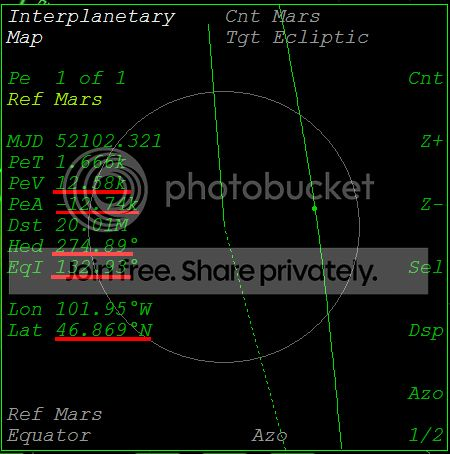Ok, we are less than 1/2 hour from periapsis, the periapsis alt is 12.74 km below the surface (we'll fix that) and the PeV is 12.58 km/s. From the heading, we can also see that we will be arriving in a retrograde direction (274.89°) and that the periapsis geo lat is going to be 46.869°N. Let's plug everything into the airspeed equation:

Airspeed = sqrt(PeV^2 + (EqRot*cos(lat))^2 - 2*PeV*(EqRot*cos(lat))*cos(90-heading)) =
sqrt(12580^2 + (240.29*cos(46.869))^2 - 2*12580*(240.29*cos(46.869))*cos(90-274.89)) = 12743.69 m/s

Now let's plug the airspeed in the static pressure equation:

STP = (2773.15 / (1.39466796e-10*airspeed^3)) = (2773.15 / (1.39466796e-10*12743.69^3)) = 9.607645 Pa

Looking up the table we can see that for Mars, that static pressure corresponds to an altitude of slightly more than 38km.
So our first action is to use the RCS thrusters and set the periapsis altitude at 38.5 km.
This will ensure that our peak hull temp will not be higher than 2500°C.
After the periapsis correction let's have another look at the Map program: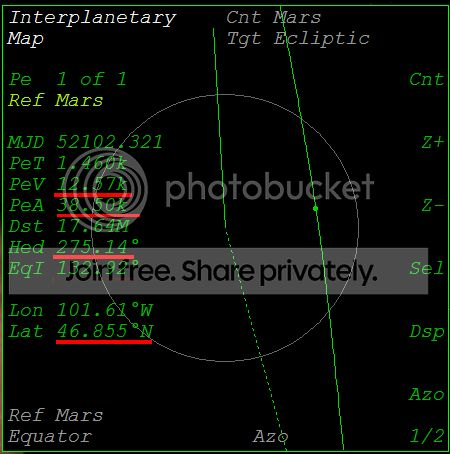The parameters changed a little bit, so let's redo the calculation. The difference should be small but we'll do it anyway for practise.

Airspeed = sqrt(12570^2 + (240.29*cos(46.855))^2 - 2*12570*(240.29*cos(46.855))*cos(90-275.14)) = 12733.67 m/s
STP = (2773.15 / (1.39466796e-10*12733.67^3)) = 9.630343 Pa

So it's pretty much the same; there is no need to change our periapsis altitude.
One thing to remember here, is that we won't instantly "pop up" inside the atmosphere at that altitude, but we'll go through the upper layers first. So our peak hull temp should be slightly less than the prediction (2500°C) for that altitude.

Let's go to periapsis and check the temperature. We start inverted at ~40° AoA and watch the vertical velocity and vertical acceleration in SurfaceMFD. Our goal is to get the vertical acceleration close to zero when we reach a vertical velocity of zero at periapsis. To do that, we'll start lowering the AoA (while inverted) when the vertical velocity is about -300 m/s.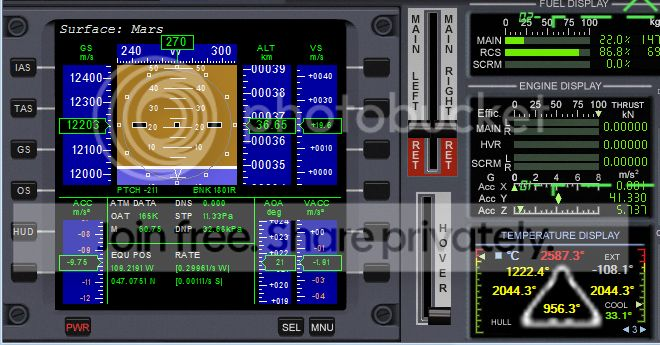Seems like we slightly overshot the periapsis. That's ok, by calculating the periapsis altitude for a hull temp of 2500°C we can afford to make such small mistakes, by giving ourselves a couple of hundred degrees room for error. Now all we need to do is hold this altitude (and drop lower as the airspeed decreases) until we are captured.
About 10 minutes later: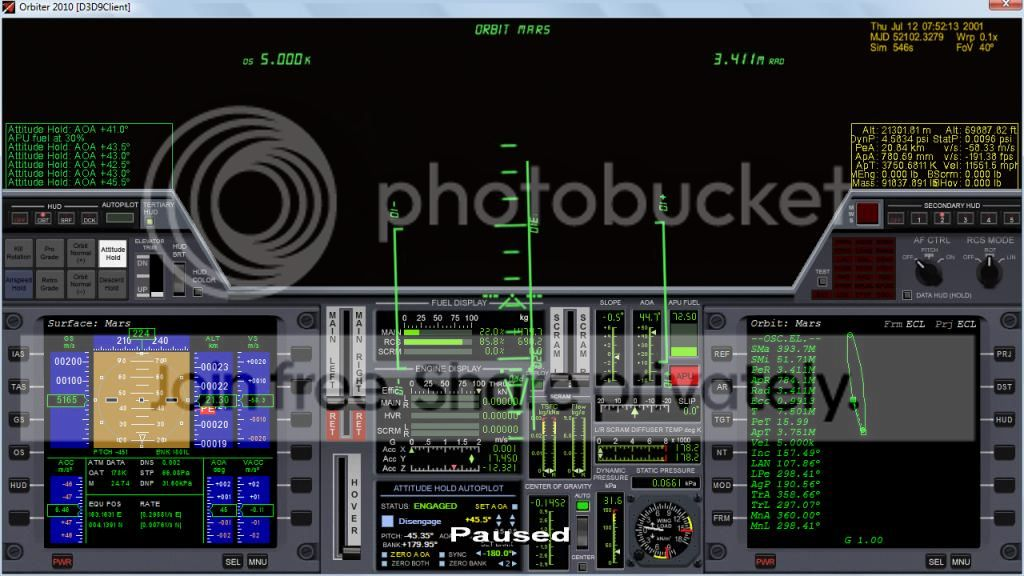Welcome to Mars orbit.
--------------------------------------------------------------------------------------------

This link will get you to a google spreadsheet that makes the hull temp calculation, so all you have to do is enter the variables from IMFD's Map program and you'll get the static pressure of the lowest altitude you can dive in for a given temperature. It's been preset to 2500°C, but you can change it if you want.
When you go to the link, select File→Create Copy so you can keep your own copy of the spreadsheet. Otherwise you won't be able to use/edit it.

One last thing to keep in mind, is that this calculation will only tell you the altutude at which you can dive inside a planet's atmosphere for a given peak hull temp. Whether you can actually perform an aerocapture at that altitude depends on other factors as well.

Have fun, happy orbiting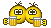Last edited:

#### SanderBuruma

##### New member
thanks for the tutorial on hull temps at different altitudes! I reckon now if we can plot the maximum altitude needed to maintain hyperbolic orbit within the atmosphere vs the temperature suffered at those altitudes you/we can know what kind of approaches are and are not viable to certain celestial bodies.

Naturally this is limited by the maximum amount of lift a spacecraft (lets say the XR2) can sustain before breaking apart due to excessive wing load and maximum temperatures by aerodynamic heat flux.

Fortunately wing load will not get near critical until after 50m/s Y-axis acceleration and this is enough to stay in inverted atmospheric hyperbolic orbits around celestial bodies with atmospheres.

#### cr1

##### Promoting vegetables in space
Hope this won't count as resurrecting an old thread. I found this post super interesting, but photobucket took a dump on the pictures, so here they are again from archive.org.

It might be more useful to edit the original post.

The atmospheric pressure is directly linked to the altitude. For most of the planets we can find the pressure for a given altitude by:(from Orbitersdk\doc\API_guide.pdf)

Let's fire up the scenario in Orbiter and open IMFD's map program:Ok, we are less than 1/2 hour from periapsis, the periapsis alt is 12.74 km below the surface (we'll fix that) and the PeV is 12.58 km/s.

After the periapsis correction let's have another look at the Map program:To do that, we'll start lowering the AoA (while inverted) when the vertical velocity is about -300 m/s.Seems like we slightly overshot the periapsis.

About 10 minutes later:Welcome to Mars orbit.